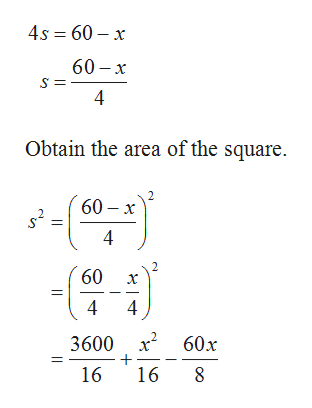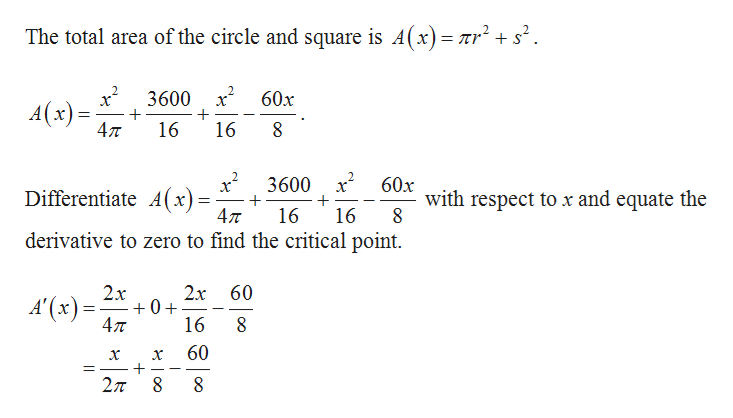# A piece of wire of length 60 is cut, and the resulting two pieces are formed to make a circle and square. What should the wire be cut to (a) maximize and (b) minimize the combined area of the circle and the square?

Question

A piece of wire of length 60 is cut, and the resulting two pieces are formed to make a circle and square. What should the wire be cut to (a) maximize and (b) minimize the combined area of the circle and the square?

check_circleExpert Solution
Step 1

Let x be the length of the wire cut for the circle then the length of the wire cut for the square.

If x be the length of the wire cut for the circle then the circumference of the circle is x.

Step 2

Let 60 – x be the length of the wire cut for the square then the perimeter of the square is 60 – x.help_outlineImage Transcriptionclose4s 60 x 60- х 4 Obtain the area of the square. 60- х 4 60 4 4 3600 2 60x 16 16 8 fullscreen
Step 3help_outlineImage TranscriptioncloseThe total area of the circle and square is A(x)= nr 3600 60x A(x) 4л 16 16 3600 x 60x with respect to x and equate the Differentiate A(x)= 4л 16 16 derivative to zero to find the critical point. 2х 60 0+ 47 2x A'(x) 16 8 60 X 27 fullscreen

### Want to see the full answer?

See Solution

#### Want to see this answer and more?

Solutions are written by subject experts who are available 24/7. Questions are typically answered within 1 hour*

See Solution
*Response times may vary by subject and question
Tagged in

### Other